# How to Make a Simple Unit Converter in Vue

BACK

March 24th, 2022, posted in Guest Posts
by Matvey Nikonorov

Here at UPDIVISION, we love to innovate. But sometimes it’s just as awesome to go back to the basics, to review some key things about our favorite technologies. So we’ve started a series of guest posts, where we invited developers from around the world to share their knowledge. To read more articles from this series, go here.

In this article, you’ll be learning how to create an energy unit converter SPA(Single Page Application) in Vue!

What You Will Learn

This is a simple, intuitive project, which makes it perfect for beginners. By building this as a Vue beginner, you will learn how to:

1. Process user input through Vue
2. Perform mathematical operations on user input and store the output as a variable
3. Manipulate the DOM(Document Object Model)
4. Use functions in Vue
5. Use buttons in Vue

The End Result

Here’s how the app you’ll be making in this tutorial will work: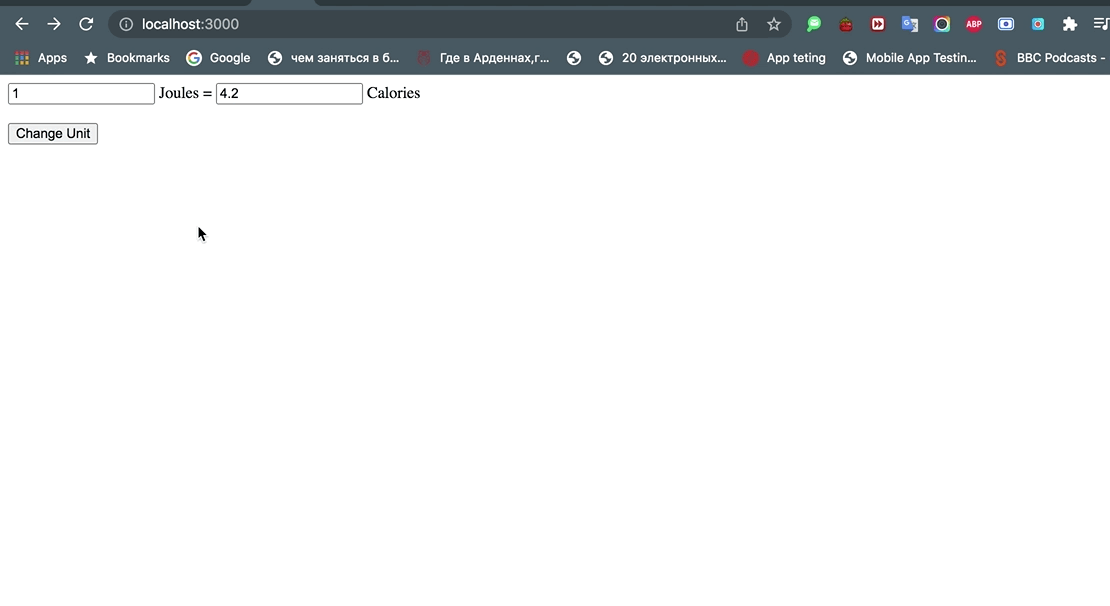Setup

First, and foremost, install the Vue CLI through npm or yarn if you haven’t already.

````bash`

`npm install -g @vue/cli`

`````

Or

````bash`

`yarn global add @vue/cli`

`````

Next, create a new Vue project by running the following.

````bash`

`npm init vue@latest`

`````

You will be presented with the following prompts:

````bash`

`✔ Project name: … <your-project-name>`

`✔ Add TypeScript? … No / Yes`

`✔ Add JSX Support? … No / Yes`

`✔ Add Vue Router for Single Page Application development? … No / Yes`

`✔ Add Pinia for state management? … No / Yes`

`✔ Add Vitest for Unit testing? … No / Yes`

`✔ Add Cypress for both Unit and End-to-End testing? … No / Yes`

`✔ Add ESLint for code quality? … No / Yes`

`✔ Add Prettier for code formating? … No / Yes`

` ````

Simply name your Vue project, and choose any additional settings if you want, but that’s not necessary. After that’s done, `cd` into your new Vue project and install npm:

````bash`

`cd <your project name>`

`````

then

````bash`

`npm install`

`````

Making a Simple Energy Unit Converter

When you open your new project’s directory in your editor of choice, you’ll see the following structure: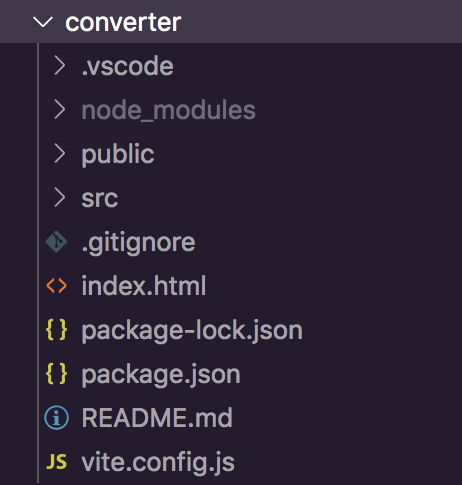Open the src directory inside your new Vue project, open the App.vue file and remove all of App.vue’s current contents so that the file is empty.

Now, to make a simple energy unit converter, first add a script section and a template section into your App.vue like so:

````vue`

` `

`<script>`

` `

`</script>`

` `

`<template>`

` `

`</template>`

` `

`````

Before we add the javascript that is going to transform units inputted by the user, we need to add input elements into the template section. They are going to pass their values to the javascript functions that are going to do the conversions.

````vue`

`<script>`

` `

`</script>`

` `

`<template>`

`<input type="number" :value="j" @change="setJoules"> Joules =`

`<input type="number" :value="kc" @change="setCalories"> Calories`

`</template>`

`````

The `:value` attribute specifies the variable in which values entered into the input elements will be stored.

Now if you run `npm run dev` in your Vue project’s directory, you should see the following terminal output: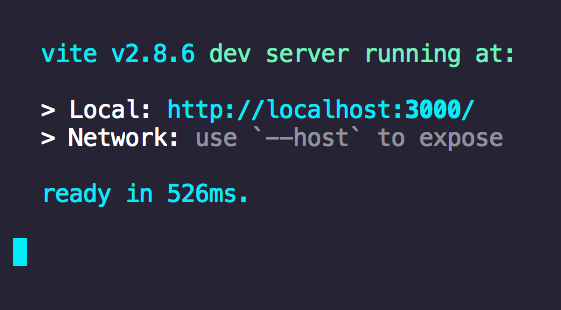Open a browser tab on the localhost specified in the terminal output and you should see the input elements displayed by your Vue app: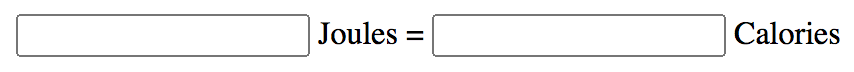You’ll soon notice that if you enter values into the input elements, nothing happens. So, let’s add the javascript to make the unit converter work!

````vue`

`export default {`

`    data() {`

`        return {`

`        }`

`    }`

`}`

`````

Inside the `data()` function, we will define the variables that we’ll be using in our energy unit converter as well as give them their default values.

````vue`

`export default {`

`    data() {`

`        return {`

`            j: 1,`

`            kc: 4.2`

`        }`

`    },`

`}`

`````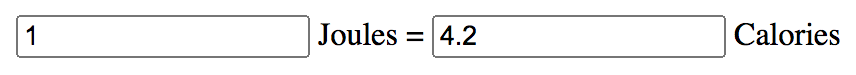Now, to make the functions that are going to convert values from the input elements, add a `methods` sub-section into your script section under the `data` sub-section like so:

````vue`

`export default {`

`    data() {`

`        return {`

`            j: 1,`

`            kc: 4.2`

`        }`

`    },`

`    methods: {`

`    }`

`}`

`````

Now it’s time to add the javascript functions that are going to convert values entered into the input elements. Each input element takes in different unit types. In this example, the first input element takes in Joules while the second one takes in Calories.

You’ve probably noticed that each input element references a function through a `@change` attribute. These functions will take in the values entered into the input elements stored in the `j` and `kc` variables and convert them into the unit represented by the other input element.

Let’s define the first input element’s function: setJoules. Here’s how it’s going to look:

````vue`

`setJoules(e, j = +e.target.value) {`

`    this.j = j`

`    this.kc = (j*4.2).toFixed(2)`

`},`

`````

Here’s what this function does:

1. It takes in the `j` variable.
2. It defines the `j` variable.
3. It sets the variable associated with the other input element(kc) as `j*4.2` and rounds the value to 2 decimal places.

Now here’s how the function referenced by the other input element is going to look:

````vue`

`setCalories(e, kc = +e.target.value) {`

`    this.kc = kc`

`    this.j = (kc/4.2).toFixed(2)`

`},`

`````

As you can see, it works similarly to the first function but this time with the `kc` variable.

Here’s how your `App.vue` should look so far:

````vue`

`<script>`

`export default {`

`    data() {`

`        return {`

`            j: 1,`

`            kc: 4.2`

`        }`

`    },`

`    methods: {`

`        setJoules(e, j = +e.target.value) {`

`            this.j = j`

`            this.kc = (j*4.2).toFixed(2)`

`        },`

`        setCalories(e, kc = +e.target.value) {`

`            this.kc = kc`

`            this.j = (kc/4.2).toFixed(2)`

`        },`

`    }`

`}`

`</script>`

` `

`<template>`

`<input type="number" :value="j" @change="setJoules"> Joules =`

`<input type="number" :value="kc" @change="setCalories"> Calories`

`</template>`

`````

Now if you open your Vue app in a browser, you will see that your input elements are able to convert their inputted values: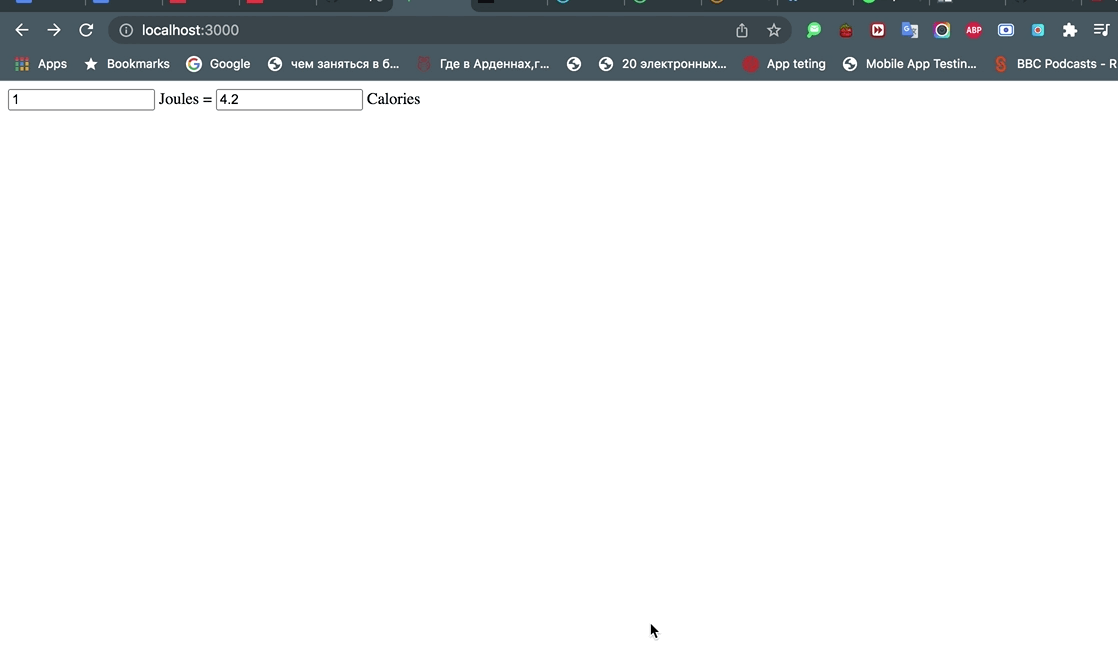As you can see, your app can convert values from Joules to Calories and vice-versa!

Changing the Input Elements

This little app is quite useful when you want to convert Joules to Calories and vice versa, but what if you want the possibility to convert Joules to another value? In this case, we’ll need to introduce another input element. For this energy converter app, let’s add an input element that will take in and display values in KWh(Kilowatt Hours), another popular measurement of energy:

````vue`

`<input type="number" :value="kwh" @change="setKWh"> KWh`

`````

`We need to put this input element inside of the following div element:`

````vue`

`<div v-if="b1 == 1" style="display: inline-block;">`

`</div>`

`````

`Then we need to put our existing “Calories” input element into the following div:`

````vue`

`<div v-if="b1 == 0" style="display: inline-block;">`

`</div>`

`````

You will see why this is necessary in a minute.

We will then have to define the `kwh` variable inside the `data()` function:

````vue`

`data() {`

`    return {`

`        j: 1,`

`        kc: 4.2,`

`        kwh: 0`

`    }`

`},`

`````

Next, you’ll need to create the `setKWh()` function:

````vue`

`setKWh(e, kwh = +e.target.value) {`

`    this.kwh = kwh`

`    this.j = (kwh/(2.777778*10**-7)).toFixed(2)`

`},`

` `

`````

Finally, you’ll need to add the following method into the `setJoules()` function:

````vue`

`setJoules(e, j = +e.target.value) {`

`    this.j = j`

`    this.kc = (j*4.2).toFixed(2)`

`    this.kwh = (j*(2.777778*10**-7)).toFixed(10)`

`},`

`````

1 KWh is 3600000 joules, a pretty large difference, so in order for the conversions to be accurate, we need to round the value in KWh to 10 decimal places.

Here’s how your `App.vue` should look like so far:

````vue`

`<script>`

`export default {`

`    data() {`

`        return {`

`            j: 1,`

`            kc: 4.2,`

`            kwh: 0`

`        }`

`    },`

`    methods: {`

`        setJoules(e, j = +e.target.value) {`

`            this.j = j`

`            this.kc = (j*4.2).toFixed(2)`

`            this.kwh = (j*(2.777778*10**-7)).toFixed(10)`

`       },`

`        setCalories(e, kc = +e.target.value) {`

`            this.kc = kc`

`            this.j = (kc/4.2).toFixed(2)`

`        },`

`       setKWh(e, kwh = +e.target.value) {`

`            this.kwh = kwh`

`            this.j = (kwh/(2.777778*10**-7)).toFixed(2)`

`        }`

`    }`

`}`

`</script>`

` `

`<template>`

`<input type="number" :value="j" @change="setJoules"> Joules =`

`<div v-if="b1 == 0" style="display: inline-block;">`

`    <input type="number" :value="kc" @change="setCalories"> Calories`

`</div>`

`<div v-if="b1 == 1" style="display: inline-block;">`

`    <input type="number" :value="kwh" @change="setKWh"> KWh`

`</div>`

`</template>`

`````

By looking at the divs containing the input elements, you’re probably wondering what the `v-if` attribute does. The answer is pretty simple: the `v-if` attribute sets a condition for displaying elements. For example, the first container div only renders its input element when `b1 == 0` while the second div only renders its input element when `b1 == 1`. The purpose of this is to give the user the ability to switch between input elements (Calories and KWh) by changing the `b1` variable.

How are they going to do that? Using this button(the br elements put the button underneath the inputs to look neater):

````vue`

`<br><br>`

`<button @click="setB">`

`    Change Unit`

`</button>`

`````

`Which when clicked, will trigger the `setB()` function through the `@click` attribute:`

````vue`

`setB(){`

`    if(this.b1 < 1){`

`        this.b1 += 1`

`    }`

`    else{`

`        this.b1 = 0`

`    }`

`}`

` `

`````

As you can see, the `setB()` function increments `b1` when its value is lower than 1, and sets its value to 0 when its value is 1.

Don’t forget to define the `b1` variable and set it’s default value to “0” so that the “Calories” input element gets rendered by default:

````vue`

`data() {`

`    return {`

`        j: 1,`

`        kc: 4.2,`

`        kwh: 0,`

`        b1: 0`

`    }`

`},`

`````

Here’s how your App.vue should look so far:

````vue`

`<script>`

`export default {`

`    data() {`

`        return {`

`            j: 1,`

`            kc: 4.2,`

`            kwh: 0,`

`            b1: 0`

`        }`

`    },`

`    methods: {`

`        setJoules(e, j = +e.target.value) {`

`            this.j = j`

`            this.kc = (j*4.2).toFixed(2)`

`            this.kwh = (j*(2.777778*10**-7)).toFixed(10)`

`       },`

`        setCalories(e, kc = +e.target.value) {`

`            this.kc = kc`

`            this.j = (kc/4.2).toFixed(2)`

`        },`

`       setKWh(e, kwh = +e.target.value) {`

`            this.kwh = kwh`

`            this.j = (kwh/(2.777778*10**-7)).toFixed(2)`

`        },`

`        setB(){`

`            if(this.b1 < 1){`

`                this.b1 += 1`

`            }`

`            else{`

`                this.b1 = 0`

`            }`

`        }`

`    }`

`}`

`</script>`

` `

`<template>`

`<input type="number" :value="j" @change="setJoules"> Joules =`

`<div v-if="b1 == 0" style="display: inline-block;">`

`    <input type="number" :value="kc" @change="setCalories"> Calories`

`</div>`

`<div v-if="b1 == 1" style="display: inline-block;">`

`    <input type="number" :value="kwh" @change="setKWh"> KWh`

`</div>`

`<br><br>`

`<button @click="setB">`

`    Change Unit`

`</button>`

`</template>`

`````

Now if you open your Vue app in a browser, you should be able to play around with your working energy unit converter app:Conclusion

Congratulations! Now you have a functioning unit converter. You can add more units into your app by following what I’ve shown with the `Calories` and `KWh` inputs.

Using what’s shown in this article, you can also make a converter around other unit types such as: distance, mass and temperature.

I hope that you found this beginner Vue project useful and fun!